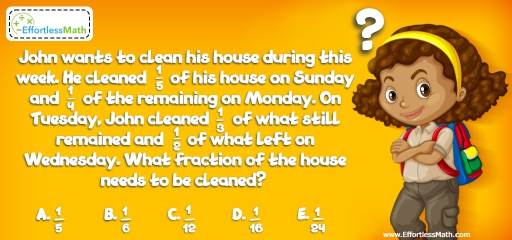# Algebra Puzzle – Challenge 49

A great math brain teaser and puzzle for smart ones! Try this math challenge to boost your brainpower with scientific exercises!## Challenge:

John wants to clean his house during this week. He cleaned $$\frac{1}{5}$$ of his house on Sunday and $$\frac{1}{4}$$ of the remaining on Monday. On Tuesday, John cleaned $$\frac{1}{3}$$ of what still remained and $$\frac{1}{2}$$ of what left on Wednesday. What fraction of the house needs to be cleaned?

A- $$\frac{1}{5}$$

B- $$\frac{1}{6}$$

C- $$\frac{1}{12}$$

D- $$\frac{1}{16}$$

E- $$\frac{1}{24}$$

### The Absolute Best Book to Challenge Your Smart Student!

John cleaned $$\frac{1}{5}$$ of his house on Sunday and $$\frac{1}{4}$$ of the remaining on Monday.
The remaining on Monday was $$\frac{4}{5}$$. Therefore, $$\frac{1}{4}$$ of $$\frac{4}{5}$$ equals:
$$\frac{1}{4} × \frac{4}{5} = \frac{4}{20} = \frac{1}{5}$$. So, he cleaned $$\frac{1}{5}$$ of his house on Sunday and another $$\frac{1}{5}$$ of his house on Monday. The remaining for the next day is $$\frac{3}{5}$$ of the house.
On Tuesday, John cleaned $$\frac{1}{3}$$ of what still remained. $$\frac{1}{3}$$ of $$\frac{3}{5}$$ of the house equals:
$$\frac{1}{3} × \frac{3}{5} = \frac{3}{15} = \frac{1}{5}$$. So far, he has cleaned $$\frac{3}{5}$$ of the house and $$\frac{2}{5}$$ of the house is left.
He cleaned $$\frac{1}{2}$$ of what left ($$\frac{2}{5}$$ of the house) on Wednesday. $$\frac{1}{2}$$ of $$\frac{2}{5}$$ of the house equals:
$$\frac{1}{2} × \frac{2}{5} =\frac{2}{10} = \frac{1}{5}$$.
Therefore, $$\frac{1}{2}$$ of the house needs to be cleaned.

The Absolute Best Books to Ace Algebra

### What people say about "Algebra Puzzle – Challenge 49 - Effortless Math: We Help Students Learn to LOVE Mathematics"?

No one replied yet.

X
45% OFF

Limited time only!

Save Over 45%

SAVE $40 It was$89.99 now it is \$49.99